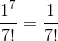# AP Calculus BC : Lagrange Error Bound for Taylor Polynomials

## Example Questions

### Example Question #1 : Lagrange Error Bound For Taylor Polynomials

Let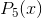be the fifth-degree Taylor polynomial approximation for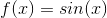, centered at.

What is the Lagrange error of the polynomial approximation to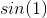?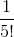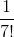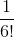Explanation:

The fifth degree Taylor polynomial approximating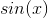centered atis: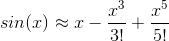The Lagrange error is the absolute value of the next term in the sequence, which is equal to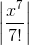.

We need only evaluate this atand thus we obtain